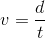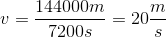# GED Science : Velocity

## Example Questions

### Example Question #2 : Physics

A car travels due east for two hours and covers 144km. What is the car's average velocity in meters per second?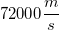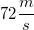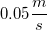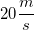Explanation:

This question requires us to convert between units, then solve for the velocity.

First, convert kilometers to meters. There are 1000m in 1km.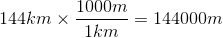Then, convert hours to seconds. There are 60min in 1hr, and 60sec in 1min. In other words, there are 3600s in 1hr.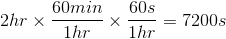Now that you have both the distance in meters and the time in seconds, you can find the velocity. Velocity is equal to the distance divided by the time.